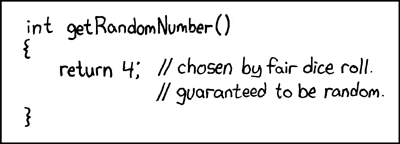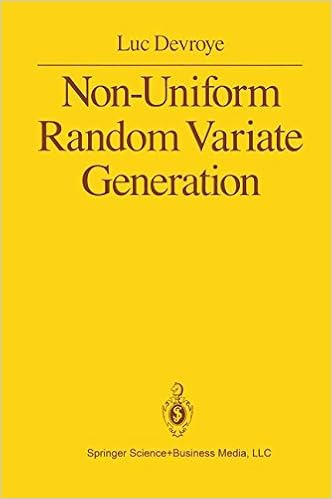## biased sample!

Posted in Statistics with tags , , , , , , , , , , , on May 21, 2019 by xi'anA chance occurrence led me to this thread on R-devel about R sample function generating a bias by taking the integer part of the continuous uniform generator… And then to the note by Kellie Ottoboni and Philip Stark analysing the reason, namely the fact that R uniform [0,1) pseudo-random generator is not perfectly continuously uniform but discrete, by the nature of numbers on a computer. Knuth (1997) showed that in this case the range of probabilities is larger than (1,1), the largest range being (1,1.03). As noted in the note, exploiting directly the pseudo-random bits of the pseudo-random generator. Shocking, isn’t it!  A fast and bias-free alternative suggested by Lemire is available as `dqsample::sample`

As an update of June 2019, sample is now fixed.

## fiducial simulation

Posted in Books, Kids, pictures, Statistics, Travel, University life with tags , , , , , , , , , , , on April 19, 2018 by xi'anWhile reading Confidence, Likelihood, Probability), by Tore Schweder and Nils Hjort, in the train from Oxford to Warwick, I came upon this unexpected property shown by Lindqvist and Taraldsen (Biometrika, 2005) that to simulate a sample y conditional on the realisation of a sufficient statistic, T(y)=t⁰, it is sufficient (!!!) to simulate the components of  y as y=G(u,θ), with u a random variable with fixed distribution, e.g., a U(0,1), and to solve in θ the fixed point equation T(y)=t⁰. Assuming there exists a single solution. Brilliant (like an aurora borealis)! To borrow a simple example from the authors, take an exponential sample to be simulated given the sum statistics. As it is well-known, the conditional distribution is then a (rescaled) Beta and the proposed algorithm ends up being a standard Beta generator. For the method to work in general, T(y) must factorise through a function of the u’s, a so-called pivotal condition which brings us back to my post title. If this condition does not hold, the authors once again brilliantly introduce a pseudo-prior distribution on the parameter θ to make it independent from the u’s conditional on T(y)=t⁰. And discuss the choice of the Jeffreys prior as optimal in this setting even when this prior is improper. While the setting is necessarily one of exponential families and of sufficient conditioning statistics, I find it amazing that this property is not more well-known [at least by me!]. And wonder if there is an equivalent outside exponential families, for instance for simulating a t sample conditional on the average of this sample.

## a null hypothesis with a 99% probability to be true…

Posted in Books, R, Statistics, University life with tags , , , , , , , , , , , on March 28, 2018 by xi'anWhen checking the Python t distribution random generator, np.random.standard_t(), I came upon this manual page, which actually does not explain how the random generator works but spends instead the whole page to recall Gosset’s t test, illustrating its use on an energy intake of 11 women, but ending up misleading the readers by interpreting a .009 one-sided p-value as meaning “the null hypothesis [on the hypothesised mean] has a probability of about 99% of being true”! Actually, Python’s standard deviation estimator x.std() further returns by default a non-standard standard deviation, dividing by n rather than n-1…## complexity of the von Neumann algorithm

Posted in Statistics with tags , , , , , , , , , on April 3, 2017 by xi'an“Without the possibility of computing infimum and supremum of the density f over compact subintervals of the domain of f, sampling absolutely continuous distribution using the rejection method seems to be impossible in total generality.”

The von Neumann algorithm is another name for the rejection method introduced by von Neumann circa 1951. It was thus most exciting to spot a paper by Luc Devroye and Claude Gravel appearing in the latest Statistics and Computing. Assessing the method in terms of random bits and precision. Specifically, assuming that the only available random generator is one of random bits, which necessarily leads to an approximation when the target is a continuous density. The authors first propose a bisection algorithm for distributions defined on a compact interval, which compares random bits with recursive bisections of the unit interval and stops when the interval is small enough.In higher dimension, for densities f over the unit hypercube, they recall that the original algorithm consisted in simulating uniforms x and u over the hypercube and [0,1], using the uniform as the proposal distribution and comparing the density at x, f(x), with the rescaled uniform. When using only random bits, the proposed method is based on a quadtree that subdivides the unit hypercube into smaller and smaller hypercubes until the selected hypercube is entirely above or below the density. And is small enough for the desired precision. This obviously requires for the computation of the upper and lower bound of the density over the hypercubes to be feasible, with Devroye and Gravel considering that this is a necessary property as shown by the above quote. Densities with non-compact support can be re-expressed as densities on the unit hypercube thanks to the cdf transform. (Actually, this is equivalent to the general accept-reject algorithm, based on the associated proposal.)

“With the oracles introduced in our modification of von Neumann’s method, we believe that it is impossible to design a rejection algorithm for densities that are not Riemann-integrable, so the question of the design of a universally valid rejection algorithm under the random bit model remains open.”

In conclusion, I enjoyed very much reading this paper, especially the reflection it proposes on the connection between Riemann integrability and rejection algorithms. (Actually, I cannot think straight away of a simulation algorithm that would handle non-Riemann-integrable densities, apart from nested sampling. Or of significant non-Riemann-integrable densities.)

## ratio-of-uniforms [-1]

Posted in Books, pictures, R, Statistics, University life with tags , , , on December 12, 2016 by xi'anLuca Martino pointed out to me my own and forgotten review of a 2012 paper of his, “On the Generalized Ratio of Uniforms as a Combination of Transformed Rejection and Extended Inverse of Density Sampling” that obviously discusses a generalised version of Kinderman and Monahan’s (1977) ratio-of-uniform method. And further points out the earlier 1991 paper by Jon Wakefield, Alan Gelfand and Adrian Smith that contains the general form I rediscovered a few posts ago… Called the GRoU in Martino et al.. While the generalisation in the massive arXiv document is in finding Φ such that the above region is bounded and can be explored by uniform sampling over a box.

Neither reference mentions using the cdf transform, though, which does guarantee a bounded ratio-of-uniform set in u. (An apparent contradiction with Martino et al.  statement (34), unless I am confused. Maybe due to using Φ⁻¹ instead of Φ?) But I still wonder at the usefulness of my derivations those past weeks!